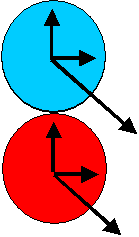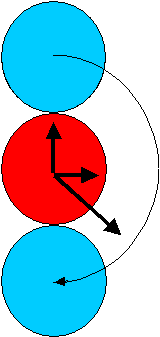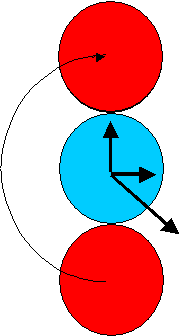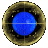Einstein's Solution to the Coin Problem
Its too late to interview Einstein, but lets try and apply the methodology Einstein used in both his Special and General Theory of Relativity. The methodology of applying a space-time reference frame (X,Y,Z,T) to disparate components of a physical system.

So here is Einstein's attack on the Coin Problem -- ignoring time considerationsFix a reference frame in the centre of both the blue AND the red coins. Call the diameter of a coin D. Then in both cases set the z-axis perpendicular to the plane of the coin, and make the x-axes horizontal (to the screen).

 Look at the situation from both reference frames:From the red reference frame perspective: The blue coin centre is initially at (0,D,0) and the blue coin rolls about the red until its centre is at (0,-D,0), and its point of contact moves from (0, D/2, 0) to (0, -D/2. 0). Meanwhile in the blue reference frame:: The red coin centre is initially at (0,-D,0), and rolls about the blue until its centre is at (0,D,0), and its point of contact moves from (0, -D/2, 0) to (0, D/2 0). That is, on both coins the point of contact has moved through 180 degrees, so that one coin is sitting on top of the other, both with the same heading as before.

A different turn of phrase is:
that just as the blue coin rolls around through half its circumference to reach the bottom of the red coin, then from the perspective of the blue coin, the red coin is rolling about it through half its circumference, so is now on the opposite of the blue coin.

I confess that as presented here, Einstein's Solution is very close to the Reciprocity/Symmetry Solution.Newtonian Differential motivated attack on the Coin ProblemHeuristic Process motivated attack on the Coin ProblemReciprocity/Symmetry solution to the Coin ProblemHomepage for the Coin ProblemReturn To Dragons Homepage

Some further questions:

• Can this argument be made rather more true to Einstein's exposition style ?
• Is there really a significant difference between an Einstein Relativity approach and the Newtonian Reciprocity approach?
• Why can/can't one take over this argument to deal with the Twin Paradox?